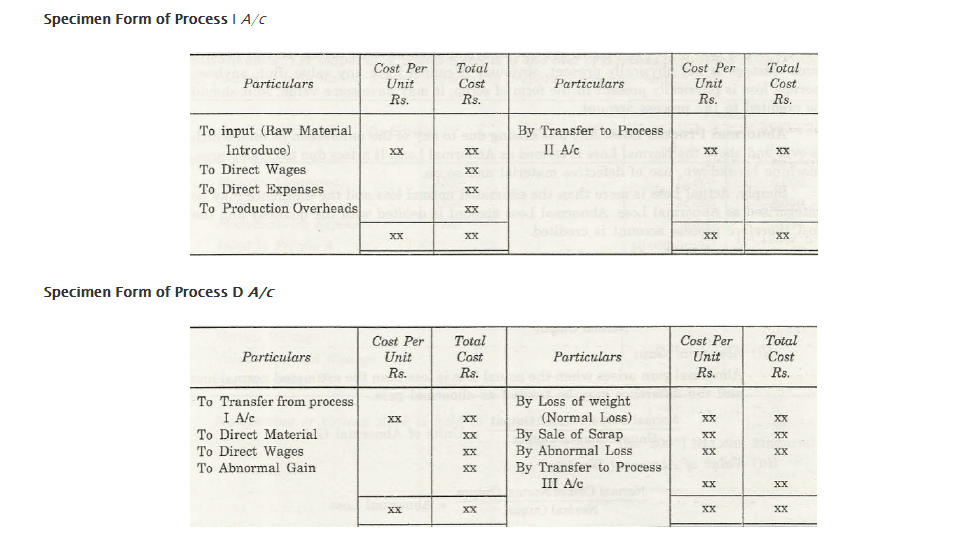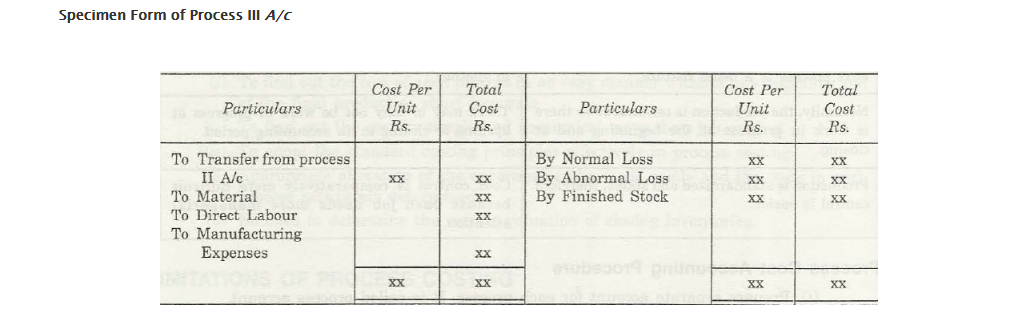Process Costing

The detection of the total cost of products at individual production process or phases is stated as process costing.

Job Costing

The method of determining the costings involved in various trades in a business house is found out by job costing. Separately funds are gathered and used in diverse projects and industrial operations under this procedure.

Differential Points between Process Costing and Job Costing

1. Process Costing

The production process follows an uninterrupted flow and the products manufactured are in uniform order in process costing.

Job costing

In job costing,the process procedure in commodity production does not follow any particular order.

1. Process Costing

There is an identity loss of product as various processes are interlinked with each other.

Job costing

Each product is distinct as the processes are unrelated and are not subjected to the authority of any other process.

1. Process Costing

When a certain costing session gets completed, the total estimation is calculated at the end of it.

Job costing

The cost calculation is based on the completion of a single work in one process. The format is similar to the payment method of work per assignment.

1. Process Costing

There is usually a transfer cost associated to process costing. In here the money is transferred from one process to another.

Job costing

There is no such process of cost transfer from one work to another.

1. Process Costing

The work progresses unhindered from the starting to the ending of a production.

Job costing

During accounting period the flow of progressive work may not be same at its beginning or closing.

1. Job costing

Job costing can be easily controlled because of its systematic and balanced production process.

Process Costing

Due to the requirement of excessive managerial attention needed for each work, controlling the cost is relatively a laborious job.

Accounting procedure associated to Process Cost

1. Create a process account by preparing a different account for an individual process.
2. Debt every direct and indirect expenditure in specific processes.
3. The initial process account should have the instituted raw materials.
4. Until and unless the production remains incomplete, the total fund used in one process will be taken as an initial cost and shifted to the following process.

5.A proper action should betaken if there are any usual or unusual profit and loss seen in the production process.Process Losses

There are two categories in which are divided.

1. Normal Process Loss
2. Abnormal Process Loss

Process Losses = Normal Process Loss + Abnormal Process Loss

Normal Process Loss

A definite input (raw material) percentage is determined as normal process loss. If stated in a simple way, normal process loss is an estimated loss before the beginning of production.

But there are times when one cannot understand the reason of loss. It may be due to any other operation or evaporation. In these cases, wastage does not have any physical presence and therefore do not have any value. Still, in scrap format, its value is present which gets calculated as a normal loss. So in the process account, this documented value should be credited.

Abnormal Process Loss

Any loss which occurs which has not been taken to prior consideration and which on calculation comes more than the normal loss is stated as an abnormal loss. There are certain reasons due to which such losses takes place. It may be because of:

1. Utilisation of defective material
2. Carelessness in production process
3. Breakdown of machineries and much more.

In easy words, when the calculated actual loss is more than normal loss, the dissimilarity in the losses is termed as an abnormal loss.

The cost is credited to the process account where input quantity is debited in the abnormal loss.

Evaluation of Abnormal Wastage

(Usual cost associated with Normal Output)/(Normal Output x Abnormal Loss)

Abnormal gain formulation

The difference in cost when the estimated loss is below the normal loss is defined as abnormal gain.

(Normal Cost of Normal Output)/(Units of Normal Output x Unit of Abnormal Gain)

Abnormal loss formulation

(Normal Cost related to Normal Production)/(Unit of Abnormal Loss x Normal Output)### Customer Reviews

My Homework Help
Rated 5.0 out of 5 based on 510 customer reviews at